Next: Ponomarenko Dynamos Up: Magnetohydrodynamic Fluids Previous: Slow and Fast Dynamos

# Cowling Anti-Dynamo Theorem

One of the most important results in slow, kinematic dynamo theory is credited to Cowling.The so-called Cowling anti-dynamo theorem states that:
An axisymmetric magnetic field cannot be maintained via dynamo action.
Let us attempt to prove this proposition.

We adopt standard cylindrical polar coordinates: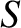. The system is assumed to possess axial symmetry, so that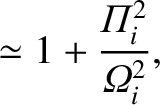. For the sake of simplicity, the plasma flow is assumed to be incompressible, which implies that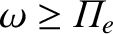.

It is convenient to split the magnetic and velocity fields into poloidal and toroidal components: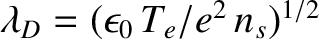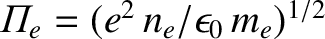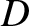(795)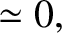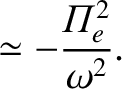(796)

Note that a poloidal vector only possesses non-zero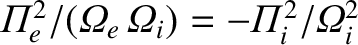- and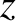-components, whereas a toroidal vector only possesses a non-zero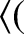-component.

The poloidal components of the magnetic and velocity fields are written: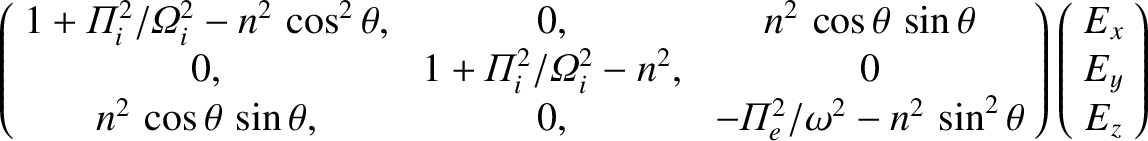(797)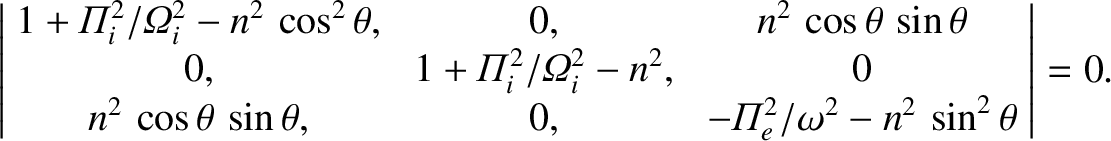(798)

where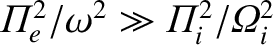and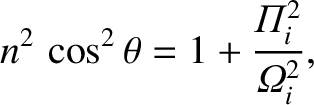. The toroidal components are given by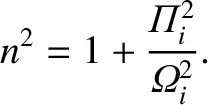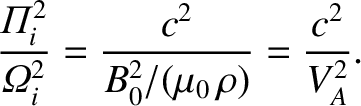(799)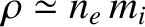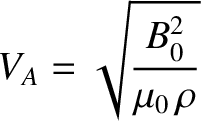(800)

Note that by writing theand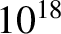fields in the above form we ensure that the constraints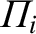andare automatically satisfied. Note, further, that since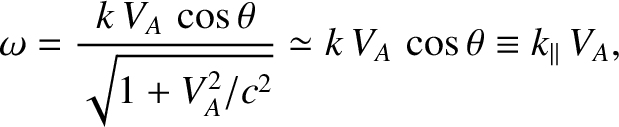and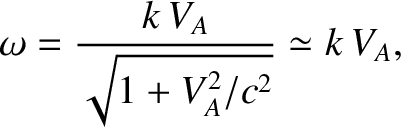, we can regard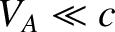and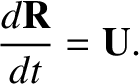as stream-functions for the magnetic and velocity fields, respectively.

The condition for the magnetic field to be maintained by dynamo currents, rather than by currents at infinity, is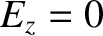(801)

where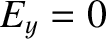. We also require the flow stream-function,, to remain bounded as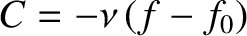.

Consider the MHD Ohm's law for a resistive plasma: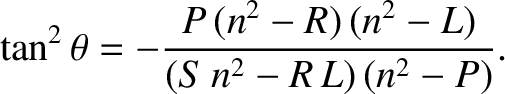(802)

Taking the toroidal component of this equation, we obtain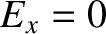(803)

It is easily demonstrated that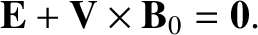(804)

Furthermore,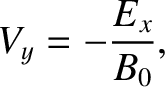(805)

and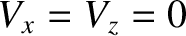(806)

Thus, Eq. (803) reduces to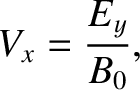(807)

Multiplying the above equation byand integrating over all space, we obtain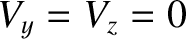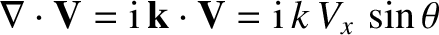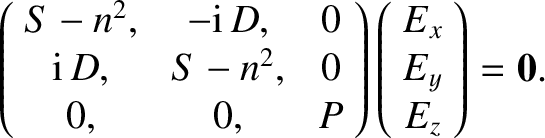(808)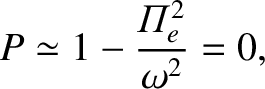The second term on the left-hand side of the above expression can be integrated by parts to give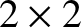(809)

where surface terms have been neglected, in accordance with Eq. (801). Likewise, the term on the right-hand side of Eq. (808) can be integrated by parts to give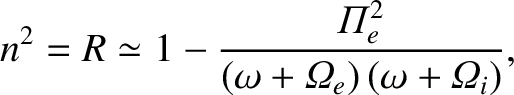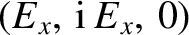(810)

Thus, Eq. (808) reduces to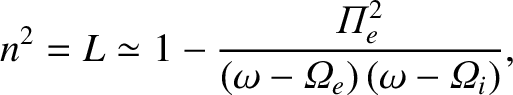(811)

It is clear from the above expression that the poloidal stream-function,, and, hence, the poloidal magnetic field,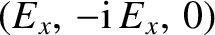, decays to zero under the influence of resistivity. We conclude that the poloidal magnetic field cannot be maintained via dynamo action.

Of course, we have not ruled out the possibility that the toroidal magnetic field can be maintained via dynamo action. In the absence of a poloidal field, the curl of the poloidal component of Eq. (802) yields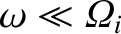(812)

which reduces to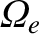(813)

Now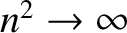(814)

and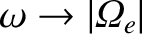(815)

Thus, Eq. (813) yields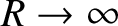(816)

where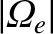(817)

Multiply Eq. (816) by, integrating over all space, and then integrating by parts, we obtain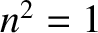(818)

It is clear from this formula that, and, hence, the toroidal magnetic field,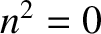, decay to zero under the influence of resistivity. We conclude that no axisymmetric magnetic field, either poloidal or toroidal, can be maintained by dynamo action, which proves Cowling's theorem.

Cowling's theorem is the earliest and most significant of a number of anti-dynamo theorems which severely restrict the types of magnetic fields which can be maintained via dynamo action. For instance, it is possible to prove that a two-dimensional magnetic field cannot be maintained by dynamo action. Here, two-dimensional'' implies that in some Cartesian coordinate system,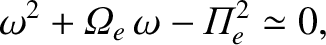, the magnetic field is independent of. The suite of anti-dynamo theorems can be summed up by saying that successful dynamos possess a rather low degree of symmetry.Next: Ponomarenko Dynamos Up: Magnetohydrodynamic Fluids Previous: Slow and Fast Dynamos
Richard Fitzpatrick 2011-03-31